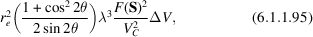International
Tables for
Crystallography
Volume C
Mathematical, physical and chemical tables
Edited by E. Prince

International Tables for Crystallography (2006). Vol. C, ch. 6.1, p. 590

## Section 6.1.1.8. Reflecting power of a crystal

E. N. Maslen,e A. G. Foxb and M. A. O'Keefec

#### 6.1.1.8. Reflecting power of a crystal

| top | pdf |

The reflecting power of a small crystal of volume ΔV, rotated at angular velocity ω through a Bragg reflection, defined as the ratio of ω times the reflected energy to the incident-beam intensity, iswhereis the unit-cell volume. This expression, which assumes negligible absorption, shows that the integrated intensity is proportional to the crystal volume. The maximum intensity is proportional to (ΔV)2, but the angular width of the reflecting region varies inversely as ΔV.

In the kinematic theory of diffraction, it is assumed that the crystal is comprised of small domains of perfect crystals for which the intensities are additive. In that case, (6.1.1.95)applies also to finite crystals.